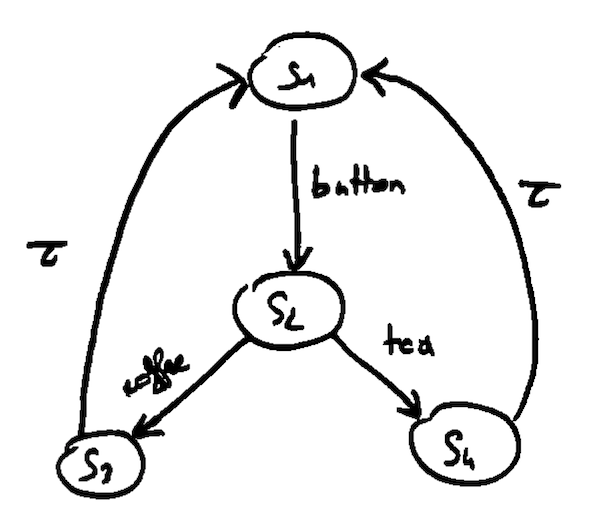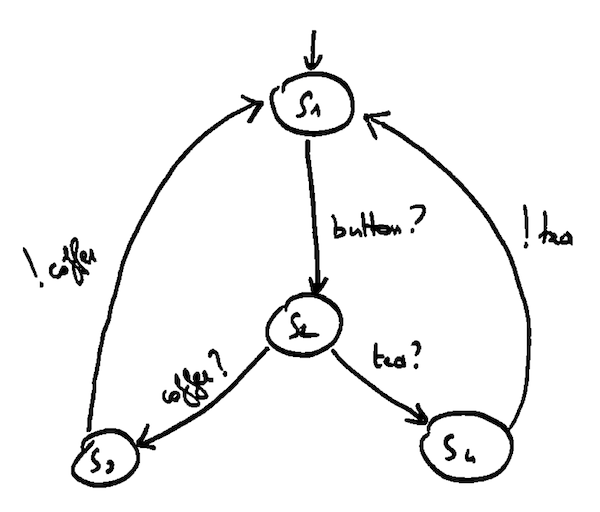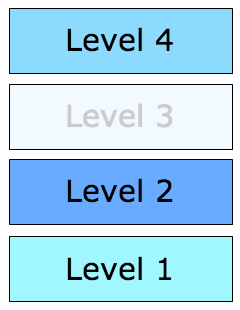# Once Upon A Time In Software Testing

William Durand - December 18, 2013

## Test Execution

Successful execution of a test case $$TC$$:

$$I_{iut}\ {\bf passes}\ TC$$

It is easily extended to a test suite $$TS$$:

$$I_{iut}\ {\bf passes}\ TS \Leftrightarrow \forall\ TC \in TS : I_{iut}\ {\bf passes}\ TC$$

$$I_{iut}\ {\bf fails}\ TC \Leftrightarrow I_{iut}\ \cancel{\bf passes} TC$$

## Example$$L = (S, Act, \rightarrow)$$
with
$$S = \lbrace s_{1}, s_{2}, s_{3}, s_{4} \rbrace$$
and
$$Act = \lbrace COFFEE, TEA, BUTTON \rbrace$$

## Example

\begin{align} traces(s_{3}) = & \lbrace \\ & BUTTON, \\ & BUTTON \cdot TEA \cdot BUTTON, \\ & \dots \rbrace = traces(s_{1}) = traces(s_{4}) \\ \\ traces(s_{2}) = & \lbrace \\ & TEA, \\ & COFFEE, \\ & TEA \cdot BUTTON \cdot TEA, \\ & \dots \rbrace \end{align}

## Input/Output LTS

By partitioning the actions labels ($$Act$$) into inputs ($$Act_{I}$$)
and outputs ($$Act_{U}$$), we can obtain an IOLTS:

$$Act = Act_{I} \cup Act_{U}$$

The names of input actions end on "$$?$$", and
those of output actions with "$$!$$".

We introduce a special action δ to denote quiescence.

## Example\begin{align} Act_{I} = & \lbrace BUTTON?, COFFEE?, TEA? \rbrace \\ Act_{U} = & \lbrace COFFEE!, TEA! \rbrace \end{align}

## Context (1/2)

Michelin relies on a method close to the Computer Integrated Manufacturing (CIM) approach to control its production: